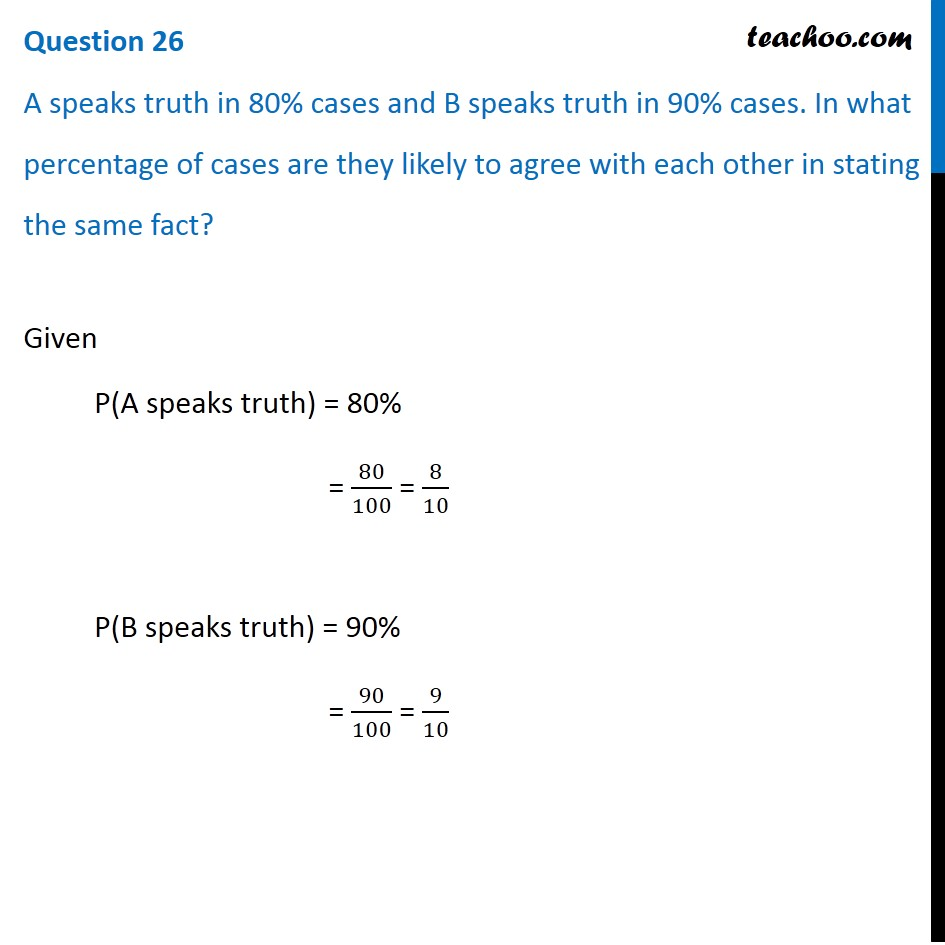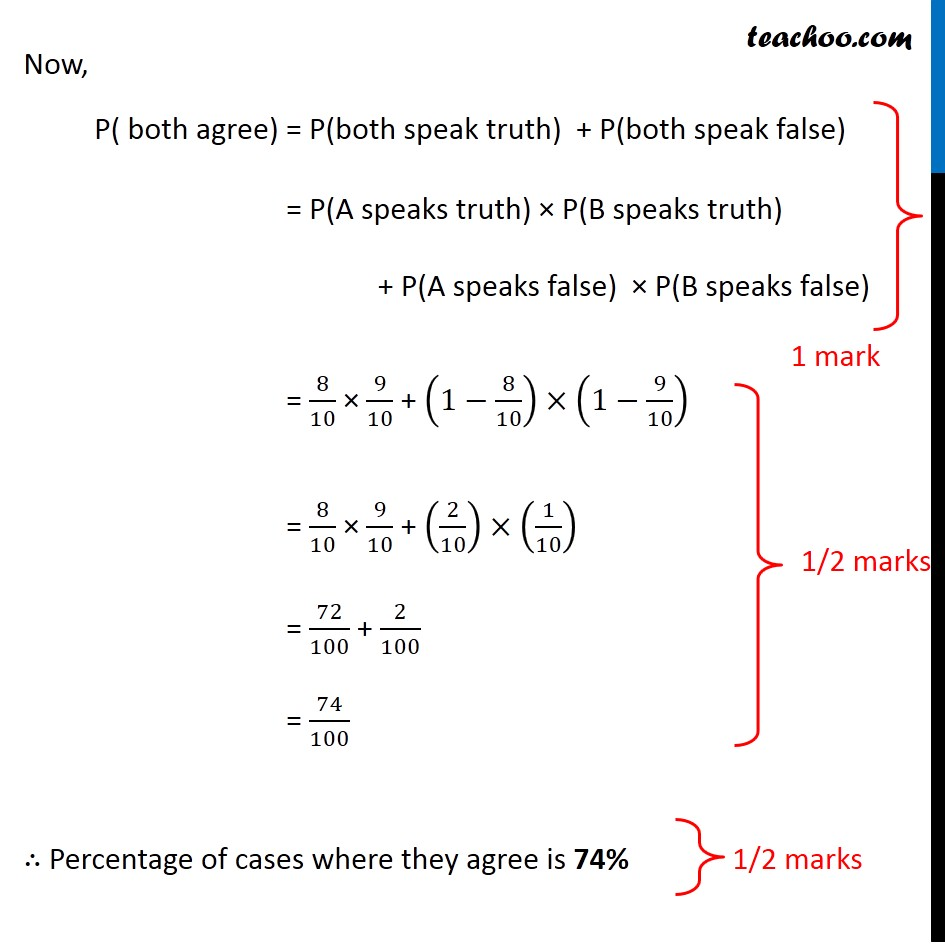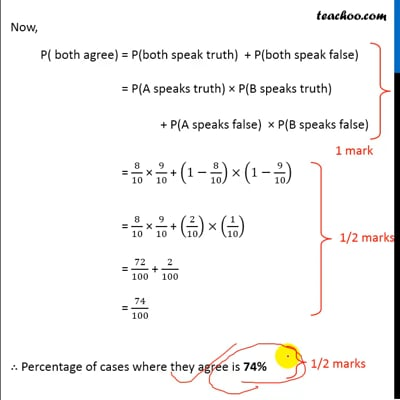CBSE Class 12 Sample Paper for 2020 Boards

Class 12
Solutions of Sample Papers and Past Year Papers - for Class 12 Boards

## A speaks truth in 80% cases and B speaks truth in 90% cases. In what percentage of cases are they likely to agree with each other in stating the same fact?This video is only available for Teachoo black users

Learn in your speed, with individual attention - Teachoo Maths 1-on-1 Class

### Transcript

Question 26 A speaks truth in 80% cases and B speaks truth in 90% cases. In what percentage of cases are they likely to agree with each other in stating the same fact? Given P(A speaks truth) = 80% = 80/100 = 8/10 P(B speaks truth) = 90% = 90/100 = 9/10 Now, P( both agree) = P(both speak truth) + P(both speak false) = P(A speaks truth) × P(B speaks truth) + P(A speaks false) × P(B speaks false) = 8/10 × 9/10 + (1−8/10)×(1−9/10) = 8/10 × 9/10 + (2/10)×(1/10) = 72/100 + 2/100 = 74/100 ∴ Percentage of cases where they agree is 74%